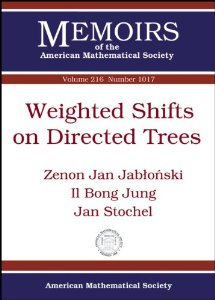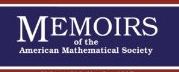## Series Monográficas: Memoirs of the AMSVol. 172. Alfsen, Erik Magnus; Shultz, Frederic W.
Non-commutative spectral theory for affine function spaces on convex sets
QA326 A52

Vol. 171. Ernest, John
Charting the operator terrain
QA329.2 E74

Vol. 96. Eisenman, Donald Alfred
Intrinsic measures on complex manifolds and holomorphic mappings
QA331 E57

Vol. 170. Ocken, Stanley
Parametrized knot theory
QA612.2 O23

Vol. 95. Folkman, Jon H.

QA612.7 F65

Vol. 169. Lazar, Aldo Joram; Taylor, D. C.
Multipliers of Pedersen's ideal
QA326 L38

Vol. 94. Auslander, Maurice; Bringer, Mark
Stable module theory
QA247.3 A87

Vol. 168. Howes, Frederick A.
Singular perturbations and differential inequalities
QA379 H67

QA274.7 K47

Vol. 167. Bismut, Jean-Michel
Théorie probabiliste du controle des diffusions
QA274.75 B57

Vol. 92. Beem, John Kelly; Woo, Peter Y.
Doubly timelike surfaces
QA611.28 B44

Vol. 91. Arsove, Maynard G.; Johnson, Guy
A conformal mapping technique for infinited connected regions
QA360 A77

Vol. 164. Cartwright, Donald I.
Extensions of positive operators between Banach lattices
QA329.2 C36

Vol. 89. Kleene, Stephen Cole

QA248.5 K534

Vol. 163. Duskin, John Williford
Simplicial methods and the interpretation of "triple" cohomology
QA169 D87

Vol. 88. Mcshane, Edward James

QA310 M32

Vol. 162. Epstein, Richard L.

QA248.54 E67

Vol. 160. Neville, Charles W.

QA333 N47

Vol. 85. Solomon, Donald William
Denjoy integration in abstract spaces
QA689 S65

Vol. 159. Lee, Chung Nim; Wasserman, Arthur
On the groups JO(G)
QA387 L43

Vol. 84. Gretsky, Neil E.
Representation theorems on Banach function spaces
QA326 G74

Vol. 83. Dauns, John

QA247 D38

Vol. 157. Leech, Jonathan
The structure of a band of groups
QA171 L485

Vol. 82. Jonah, David W.
Cohomology of coalgebras
QA612.3 J64

Vol. 156. Illman, Soren

QA612.3 I54

Vol. 155. May, J. Peter.
Classifying spaces and fibrations
QA612.6 M39

Vol. 80. Steinberg, Robert
Endomorphisms of linear agebraic groups
QA184 S74

Vol. 154. Zaslavsky, Thomas

QA167 Z37

Vol. 79. Brezin, Jonathan Paul
Unitary representation theory for solvable Lie groups
QA387 B74

Vol. 153. Millett, Kenneth C.
Piecewise linear concordances and isotopies
QA613.4 M54

Vol. 78. Moore, Robert Thorpe

QA322 M66

Vol. 152. Andrews, George E.
On the general Rogers-Ramanujan theorem
QA241 A523

Vol. 77. Rockafellar, R. Tyrrell
Monotone processes of convex and concave type
Q640 R63

Vol. 151. Murasugi, Kunio

QA612.23 M86

Vol. 76. Hale, Jack K., Meyer, Kenneth R.
A class of functional equations of neutral type
QA431 H34

Vol. 150. Thompson, Richard Leslie
Equilibrium states on thin energy shells
QC174.84 T45

Vol. 75. Effros, Edward G.; Hahn, Frank John

QA601 E44

Vol. 74. Massey, William Schumacher; Peterson, F. P.
The mod 2 cohomology structure of certain fibre spaces
QA611 M375

Vol. 72. Mahowald, Mark E.
The metastable homotopy of S'
QA611 M326

Vol. 71. Humphreys, James Edward
Algebraic groups and modular Lie algebras
QA171 H83

Vol. 70. Pierce, Richard S.
Modules over commutative regular rings
QA247 P54

Vol. 143. Donaldson, Thomas

QA379 D65

Vol. 68. Harrison, David Kent
Finite and infinite primes for rings and fields
QA246 H37

Vol. 142. Gugenheim, V. K. A. M.; May, J. Peter
On the theory ad applications of differential torsion products
QA169 G84

Vol. 67. Bernstein, Leon
The modified algorithm of Jacobi-Perron
QA9.58 B47

Vol. 141. Sanders, Jack Palmer

QA612.3 S35

Vol. 66. Berger, Robert
The undecidability of the domino problem
QA297 B47

Vol. 65. Brown, William G.

QA646 B76

Vol. 139. Thomas, Erik

QA312 T46

Vol. 64. Guillemin, Victor W.
Deformation theory of pseudogroup structures
QA641 G85

Vol. 137. Lazarov, Connor; Wasserman, Arthur

QA387 L39

Vol. 62. Auslander, Louis; Moore, Calvon C.

QA387 A86

Vol. 136. Racine, Michel L.

QA252.5 R32

Vol. 61. Prosser, Reese T.

QC174.12 P76

Vol. 135. Jackson, Robert John Victor
Canonical differential operators and lower order symbols
QA614 J3

Vol. 60. Conner, P. E.; Floyd, Edwin Earl

QA935 C65

Vol. 134. Coddington, Earl A.
Extension theory of formally normal and symmetric subspaces
QA329.2 C62

Vol. 59. Wainger, Stephen

QA404 W34

Vol. 133. Gordon, Robert; Robson, James Christopher

QA251.3 G67

Vol. 58. Cholewinski, Frank M.
A Hankel convolution complex inversion theory
QA403.5 C46

Vol. 132. Knyon, Hewitt; Morse, A. P.
Web derivatives
QA312 K45

Vol. 57. Levy, Azriel
A hierarchy of formulas in set theory
QA248 L48

Vol. 130. Pierce, Richard S.
Compact zero-dimensional metric spaces of finite type
QA611.28 P54

Vol. 55. Eilenberg, Samuel
Foundations of relative homological algebra
QA157 E5

Vol. 129. Hejhal, Dennis A.
Theta functions, kernel functions and Abelian integrals
QA345 H45

Vol. 54. Reay, John R.
Generalizations of a theorem of Carathéodory
QA640 R43

Vol. 128. Dubinsky, E.; Ramanujan, N. S.
On-nuclearity
QA322 D83

Vol. 53. Topping, David M.
QA252.5 T66

Vol. 127. Johnson, Barry Edward
Cohomology in Banach algebras
QA326 J63

Vol. 52. Chase, S. V.; Harrison, D. K.; Rosenberg, Alex
Abelian extensions of commutative rings
QA247 C42

Vol. 126. Elliott, Robert James; Kalton, Nigel J.

QA269 E55

Vol. 51. Clarke, Douglas Albert
Hierarchies of predicates of finite types
QA248.5 C53

Vol. 125. Nadler, S. B.; J. Quinn

QA611.28 N334

Vol. 124. Goodearl, K. R.
Singular torsion and the splitting properties
QA251.5 G652

Vol. 49. Matlis, Eben

QA935 M37

Vol. 48. Lindenstrauss, Joram
Extension of compact operators
QA322.2 L55

Vol. 122. Hofmann, Karl Heinrich; Keimel, Klaus
A general character theory for partially ordered sets and lattices
QA169 H64

Vol. 121. Skala, Helen

QA171.5 S53

Vol. 46. Gross, Leonard
Harmonic analysis on Hilbert space
QA403 G7

Vol. 120. Blatter, Jorg
Grothendieck spaces in approximation theory
QA221 B53

Vol. 45. Prosser, Reese T.

QA154 A5

Vol. 119. Stanley, Richard P.

QA164 S72

Vol. 44. Oglesby, Francis C.

QA9 O3

Vol. 118. QA374 D8

QA374 D8 (En el IIMAS)

Vol. 43. Hofmann, Karl Heinrich; Mostert, Paul S.
Splitting in topological groups
QC174.5 H64

Vol. 117. Mcmullen, John R.
Extensions of positive-definite functions
QA331 M33

Vol. 42. Liulevicius, Arunas Leonardas

QA611 L5

Vol. 115. Harris, Douglas
Structures in topology
QA611.24 H37

Vol. 40. Hamstrom, Mary Elizabeth

QA360 H3

Vol. 39. Shapiro, Victor L
Topics in Fourier and geometric analysis
QA331.5 S4

Vol. 38. Beamont, Ross Allen
Torsion free groups of rank two
QC1745 B4

On the mixed problem for a hyperbolic equation
QA371 B35

Vol. 37. Olum, Paul

QA611 O5

Vol. 36. Palais, Richard Sheldon

QA191 P3

Vol. 110. Bures, Donald John Charles
Abelian subalgebras of von Neumann algebras
QA345 B87

Vol. 35. Bellman, Richard; Cooke, Kenneth L.
Asymptotic behaviour of solutions of differential-difference equations
QA373 B39

Vol. 34. Zaustinsky, Eugene Michael
Spaces with non-symmetric distance
QA689 Z38

Vol. 108. Gelbart, Stephen S.
Fourier analysis on matrix space
QA404 G45

Vol. 33. Snapper, Ernest

QA611 S58

Vol. 32. Pierce, Richard S.

QA171.5 P52

Vol. 31. Loud, Warren Simms
Periodic solutions of x"+cx-'+g(x)=ef(t)
QA431 L595

Vol. 104. Faulkner, John R.
Octonion planes defined by quadratic Jordan algebras
QA252.5 F37

Vol. 29. Halpern, Edward

QA611 H33

Vol. 103. Stong, Robert E.

QA612.3 S76

Vol. 102. Miller, Richard Kermit; Sell, George R.

QA431 M55

Vol. 27. Thomas, Emery

QA611 T43

Vol. 26. Strodt, Walter Charles

QA371 S8

Vol. 100 Nagano, T.
Homotopy invariants in differential geometry
En compra

Vol. 25. Erdelyi, Arthur; Swasnson C. A.
Asymptotic forms of Whittaker's confluent hypergeometric functions
QA351 E7

Vol. 24. Namioka, Isaac

QA261 N33

QA611.5 A35

Vol. 23. Dickson, Douglas G.

QA371 D5

### ¿Quién está en línea?

Hay 139 invitados y ningún miembro en línea

Go to top